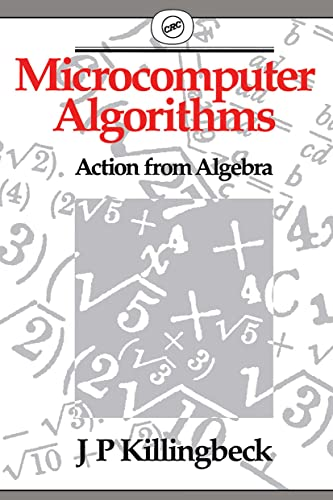# Microcomputer Algorithms by John Killingbeck (University of Hull, UK)

Out of Stock
\$19.49
inc. GST
Stresses the mathematical basis behind the use of many algorithms of computational mathematics, providing descriptions on how to generate algorithms for a large number of different uses. Covering a range of mathematical and physical applications, this book contains the theory of 25 algorithms.
Only 0 left

## Microcomputer Algorithms Summary

### Microcomputer Algorithms: Action from Algebra by John Killingbeck (University of Hull, UK)

Although the computing facilities available to scientists are becoming more powerful, the problems they are addressing are increasingly complex. The mathematical methods for simplifying the computing procedures are therefore as important as ever. Microcomputer Algorithms: Action from Algebra stresses the mathematical basis behind the use of many algorithms of computational mathematics, providing detailed descriptions on how to generate algorithms for a large number of different uses.

Covering a wide range of mathematical and physical applications, the book contains the theory of 25 algorithms. The mathematical theory for each algorithm is described in detail prior to discussing the algorithm in full, with complete program listings. The book presents the algorithms in modular form, allowing for easy interpretation, for the adaptation to readers' specific requirements without difficulty, and for use with various microcomputers.

Blending mathematics and programming in one volume, this book will be of broad interest to all scientists and engineers, particularly those physicists using microcomputers for scientific problem handling. Students handling numerical data for research projects will also find the book useful.

### Why buy from World of BooksOur excellent value books literally don't cost the earthFree delivery in AustraliaEvery used book bought is one saved from landfill

## Microcomputer Algorithms Reviews

..."a practical and valuable resource for researchers and teachers in scientific or mathematical computing who wish to use, or to explain to others, the specific and useful microprogramming style...for using small capacity computer systems more efficiently. A number of new and unorthodox methods are introduced and some algorithms have characteristics which have yet to be fully explored. This aspect will add spice to the appeal of the book to researchers in numerical methods generally." The Australian Computer Journal ractical and valuable resource for researchers and teachers in scientific or mathematical computing who wish to use, or to explain to others, the specific and useful microprogramming style...for using small capacity computer systems more efficiently. A number of new and unorthodox methods are introduced and some algorithms have characteristics which have yet to be fully explored. This aspect will add spice to the appeal of the book to researchers in numerical methods generally." The Australian Computer Journal

General introduction
Root-finding methods and their application
The Richardson extrapolation method
Some interpolation and extrapolation methods
The matrix inverse and generalized inverse
The matrix eigenvalue problem
Two perturbation methods
Finite difference eigenvalue calculations
Recurrence relation methods
Two research problems
Bibliography
Index

GOR010191718
Microcomputer Algorithms: Action from Algebra by John Killingbeck (University of Hull, UK)
John Killingbeck (University of Hull, UK)